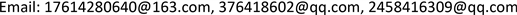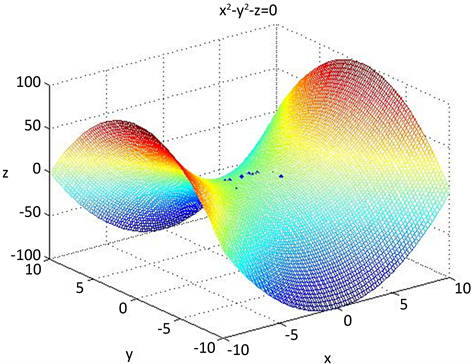﻿ 定义于马鞍面上的多元Lagrange插值 Multivariate Lagrange Interpolation Defined on Saddle Surface

Vol.06 No.09(2017), Article ID:22956,5 pages
10.12677/AAM.2017.69126

Multivariate Lagrange Interpolation Defined on Saddle Surface

Nan Zhao, Fu Liu, Lihong Cui

Liaoning Normal University, Dalian LiaoningReceived: Nov. 14th, 2017; accepted: Dec. 1st, 2017; published: Dec. 8th, 2017ABSTRACT

Based on the results of Lagrange interpolation of binary functions, we study the regularity of Lagrange interpolation nodes of ternary functions and propose the basic concepts of Lagrange interpolation regular nodes defined on saddle. By interpolating some basic theories and topological structures of regular node groups, an additive conic surface method of constructing Lagrange interpolation solvable nodes defined on the saddle surface is obtained. These methods are all constructed in a superposed manner, which creates a very convenient condition for compiling a computer algorithm program, and then automatically completing the construction of an interpolated node group and obtaining the interpolation format on the computer. Finally, an example is given to illustrate the effectiveness of the proposed algorithm.

Keywords:Saddle Surface, Multivariate Lagrange Interpolation, Regular Set of Nodes, Superposition Interpolation Method1. 引言

2. 基本定义和基本定理

$m=\left(\begin{array}{c}n+3\\ 3\end{array}\right)$ ，令 $Α={\left\{{Q}_{i}\right\}}_{i=1}^{m}$${R}^{3}$ 中m个互异点构成的点集，如果对于任意给定的数组 $\left\{{f}_{i}\in R|i=1,\cdots ,m\right\}$ ，恒存在唯一多项式 $p\left(x,y,z\right)\in {P}_{n}^{\left(3\right)}$ ，使之满足： $p\left({Q}_{i}\right)={f}_{i},i=1,\cdots ,m$ ，则称 $Α$${P}_{n}^{\left(3\right)}$ 的一个正则结点组。

$F$ 为如上所定义的马鞍面， ${P}_{n}^{\left(3\right)}\left(F\right)$${P}_{n}^{\left(3\right)}$$F$ 上的限制。 $\mathrm{dim}{P}_{n}^{\left(3\right)}\left(F\right)=\left(\begin{array}{c}n+3\\ 3\end{array}\right)-\left(\begin{array}{c}n+3-2\\ 3\end{array}\right)$$m=\mathrm{dim}{P}_{n}^{\left(3\right)}\left(F\right)$ ，称 $Α={\left\{{Q}_{i}\right\}}_{i=1}^{m}\subset F$ 为定义于 $F$ 上的一个n次插值正则结点组，如果对于任意给定的数组 $\left\{{f}_{i}\in R|i=1,\cdots ,m\right\}$ ，恒存在多项式 $p\left(x,y,z\right)\in {P}_{n}^{\left(3\right)}$ ，满足 $p\left({Q}_{i}\right)={f}_{i},i=1,\cdots ,m$$\mathrm{dim}{P}_{n}^{\left(3\right)}\left(F\right)=\left(\begin{array}{c}n+3\\ 3\end{array}\right)-\left(\begin{array}{c}n+3-2\\ 3\end{array}\right)$

$m$ 为如上所定义， $r=\left(\begin{array}{c}n+5\\ 3\end{array}\right)$ ，结点组 $Α={\left\{{Q}_{i}\right\}}_{i=1}^{m}\not\subset F$ 关于 ${P}_{n}^{\left(3\right)}$ 的一个正则结点组，而 $Β={\left\{{Q}_{i}\right\}}_{i=m+1}^{r}$ 是定义于 $F$ 的一个 $n+2$ 次正则结点组，则 ${\left\{{Q}_{i}\right\}}_{i=1}^{r}=Α\cup Β$ 必定构成 ${P}_{n+2}^{\left(3\right)}$ 的正则结点组。

$Α={\left\{{Q}_{i}\right\}}_{i=1}^{{\left(n+1\right)}^{2}}$$F$ 上的 $n$ 次插值正则结点组，平面 $p\left(x,y,z\right)\cap \text{A}=\varphi$$F$ 横截相交于圆周曲线 $C\left(x,y,z\right)$$\text{B}$ 是定义于 $C$ 上的一个 $n+1$ 次正则结点组，则 $\text{A}\cup \text{B}$ 必定构成定义于 $F$ 上的一个 $n+1$ 次正则结点组。

$F$ 上的 $m={\left(n+1\right)}^{2}$ 个互异点 ${\left\{{Q}_{i}\right\}}_{i=1}^{m}$ 能够做成定义于 $F$ 上的n次插值正则结点组的充分必要条件是，若存在 $p\left(x,y,z\right)\in {P}_{n}^{\left(3\right)}$ ，满足 $p\left({Q}_{i}\right)=0,i=1,\cdots ,m$ ，蕴含如此的 $p\left(x,y,z\right)$$F$ 恒为零。

3. 定理的证明

$p\left(x,y,z\right)={p}_{1}\left(x,y,z\right)+\left(\frac{{x}^{2}}{p}-\frac{{y}^{2}}{q}-2z\right){p}_{2}\left(x,y,z\right)$

${p}_{2}\left({Q}_{i}\right)=\frac{{f}_{i}-{p}_{1}\left({Q}_{i}\right)}{\frac{{x}_{i}^{2}}{p}-\frac{{y}_{i}^{2}}{q}-2{z}_{i}},\text{\hspace{0.17em}}i=m+1,\cdots ,r$ (2.1)

$p\left(x,y,z\right)=\stackrel{˜}{p}\left(x,y,z\right)=\left(\frac{{x}^{2}}{p}-\frac{{y}^{2}}{q}-2z\right){p}_{2}\left(x,y,z\right)\in {p}_{n}^{\left(3\right)}$

$p\left(x,y,z\right)$ 在F上恒为零值。

4. 算法

$f\left(x,y,z\right)={a}_{1}{x}^{2}+{a}_{2}{y}^{2}+{a}_{3}{z}^{2}+{a}_{4}xy+{a}_{5}xz+{a}_{6}yz+{a}_{7}x+{a}_{8}y+{a}_{9}z+{a}_{10}$

$A=\left[\begin{array}{cccccccccc}0& 1& 1& 0& 1& 0& 0& 1& 1& 1\\ 1& 2& 1& \sqrt{2}& -\sqrt{2}& -1& 1& \sqrt{2}& -1& 1\\ 1& 1& 0& 1& 0& 0& 1& 1& 0& 1\\ 1& 1& 0& -1& 0& 0& -1& 1& 0& 1\\ 2& 1& 1& \sqrt{2}& 1& \sqrt{2}& \sqrt{2}& 1& 1& 1\\ 2& 1& 1& -\sqrt{2}& 1& -\sqrt{2}& -\sqrt{2}& 1& 1& 1\\ 4& 0& 4& 0& 0& 4& 2& 0& 2& 1\\ 4& 1& 9& -2& -3& 6& 2& -1& 3& 1\\ 4& 0& 4& 0& 0& -4& -2& 0& 2& 1\\ 4& 1& 9& 2& -3& -6& -2& -1& 3& 1\end{array}\right]$ , $X=\left[\begin{array}{c}{a}_{1}\\ {a}_{2}\\ {a}_{3}\\ a{}_{4}\\ {a}_{5}\\ {a}_{6}\\ {a}_{7}\\ {a}_{8}\\ {a}_{9}\\ {a}_{10}\end{array}\right]$ , $B=\left[\begin{array}{c}\sqrt{2}\\ \sqrt{2}\\ \sqrt{2}\\ \sqrt{2}\\ 2\\ 2\\ \sqrt{8}\\ \sqrt{14}\\ \sqrt{8}\\ \sqrt{14}\end{array}\right]$Figure 1. The effect picture of saddle point taking

Multivariate Lagrange Interpolation Defined on Saddle Surface[J]. 应用数学进展, 2017, 06(09): 1045-1049. http://dx.doi.org/10.12677/AAM.2017.69126

1. 1. De Boor, C. and Ron, A. (1990) On Multivariate Polynomial Interpolation. Constructive Approximation, 6, 287-302.
https://doi.org/10.1007/BF01890412

2. 2. Liang, X.Z., Wang, R.H., Cui, L.H., et al. (2006) Some Researches on Trivariate Lagrange Interpolation. Journal of Computational and Applied Mathematics, 195, 192-205.
https://doi.org/10.1016/j.cam.2005.03.083

3. 3. 梁学章, 张明, 张慧杰, 等. 关于球面上的Lagrange插值[J]. 高等学校计算数学学报, 2011(33): 169-177.

4. 4. Castell, W.Z., Fernandez, N.L. and Xu, Y. (2007) Polynomial In-terpolation on the Unit Sphere II. Advances in Computational Mathematics, 26, 155-171
https://doi.org/10.1007/s10444-005-7510-5

5. 5. 梁学章. 关于多元函数的插值与逼近[J]. 高等学校计算数学学报, 1979(1): 123-124.

6. 6. 梁学章. 二元插值的适定结点组与迭加插值法[J]. 吉林大学自然科学学报, 1979(1): 27-32.

7. 7. 梁学章, 张洁琳, 崔利宏. 多元Lagrange插值与Cayley-Bacharach定理[J]. 高等数学计算数学学报, 2005(27): 276-281.